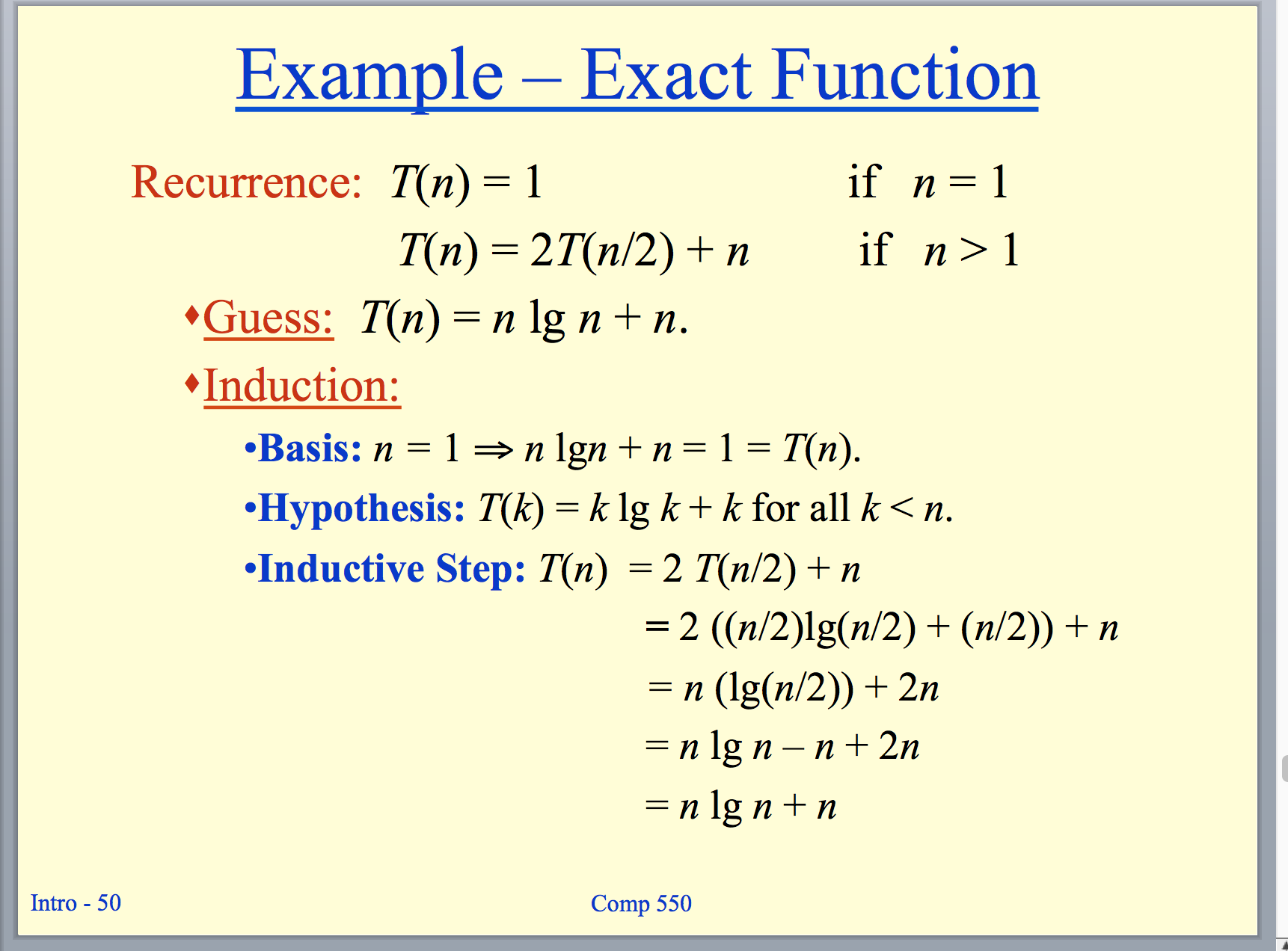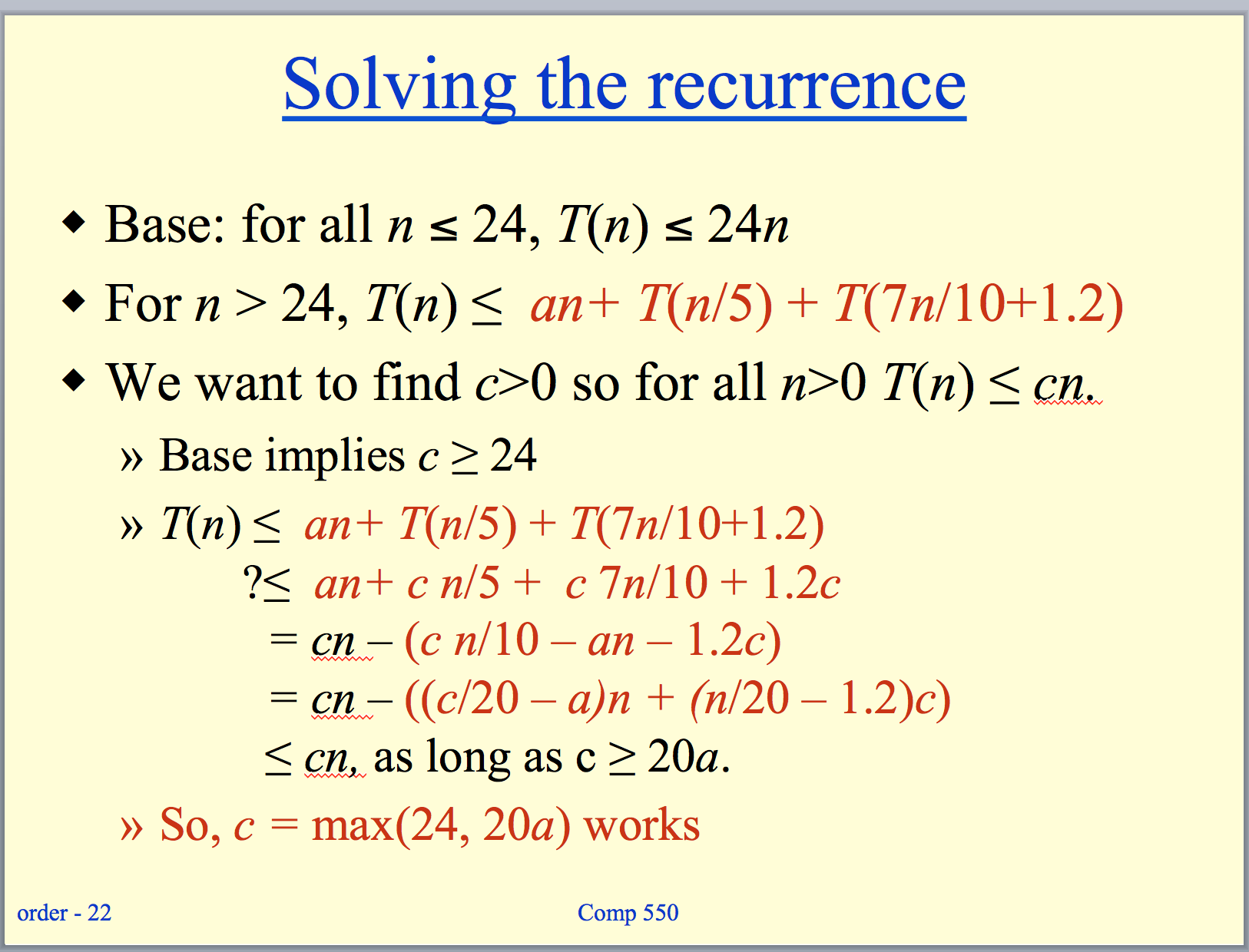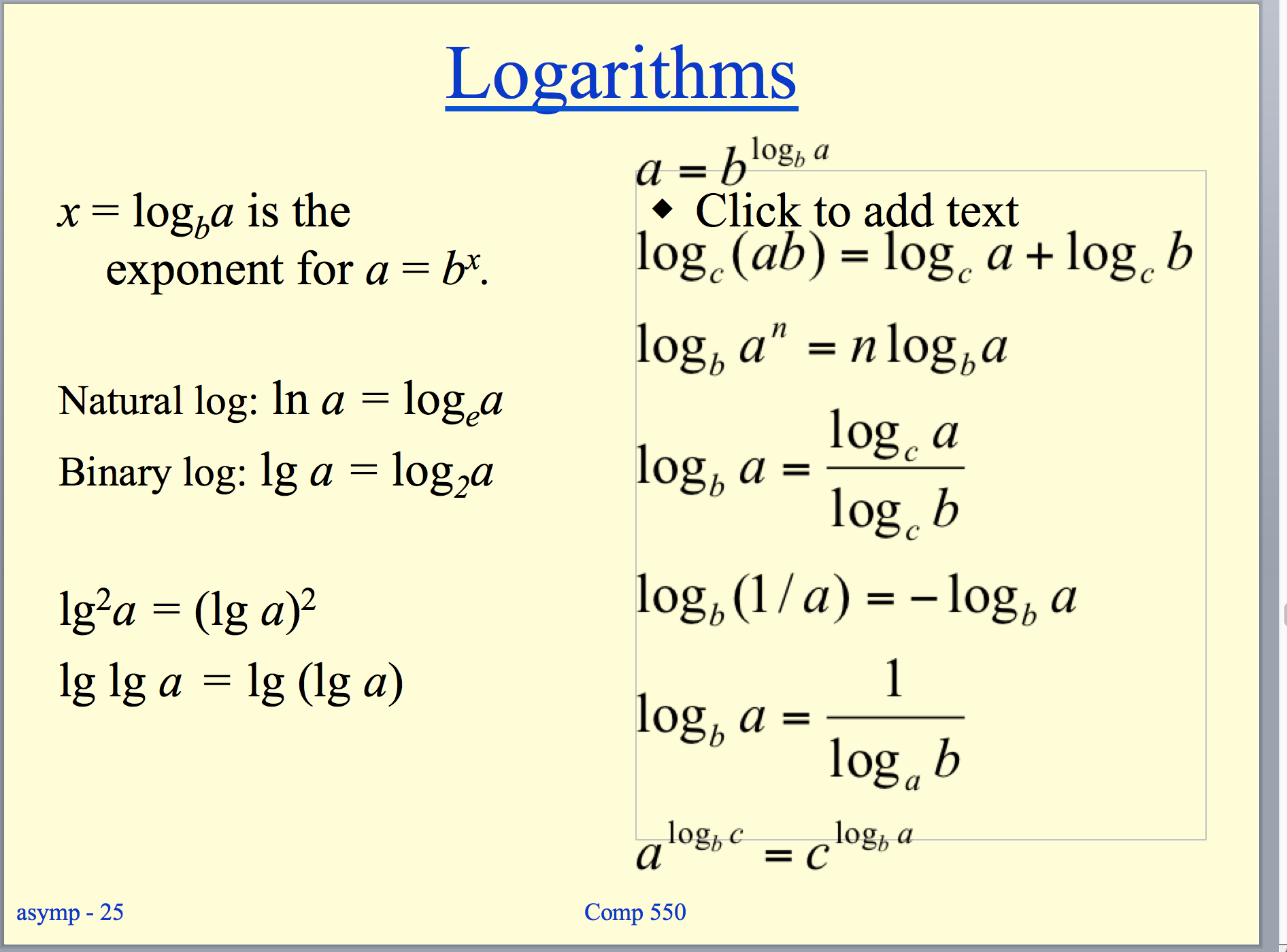```Studying for Algorithms

From the midterm:
Need to understand the log problem, 2b.

Red black trees: binary trees that are ensured balance. Take O(log n) in worst case.

Showing that a recursive function is bounded above: given the equation you’re trying to get a form of, just plug that equation in in place of T() and then try to show that you can simplify down to the appropriate form.

You can build a min/max heap in O(n) time.

Quick sort is worst when array is already sorted or reverse sorted.

Quick sort is not very efficient on small lists and is slow if there are many identical keys.

Quick sort is not stable.

Stable sorting algorithm: a sort in which equal items maintain the same relative order.

We can make quick sort run in Theta(n log n) using median finding. Can get ith largest element in theta(n) worst case using median.

Randomized quick sort is no different best/worse case than quicksotr.

Randomized Quick sort is better than quicksort for preordered input.

Asymptotic bounds refer to O, Omega, etc.

For expected value problems, you could try writing out the possible cases, and see what the expected value might be.

Insertion sort: Like one sorts cards. Consider looking through the cards one by one from left to right, and then any time you see something that should be lower you move all the cards up and put that card in the correct spot.

Merge sort: Recursive call to merge sort on two halves of the call, and at the end a call to merge which will actually do the work for us of combining sorts.

Quick sort: (again recursive) You can have non-random and random. In non-random, you choose the last element to be the pivot, and then you organize everything into what’s less than and what’s greater than the pivot and then once you’ve compared everything you put the pivot right between those two groups. Then, you call again on the two groups, those greater and those less than the pivot. The loop invariants are that everything below is in one area, above is in another, and pivot is certain index.

Heap sort: Put all the elements into a binary tree, and then pull them out one by one?

Write down all of the pseudocode for the sorting algorithms.

Could draw out recursion tree.

Guess method:￼￼

Logarithms:￼
```